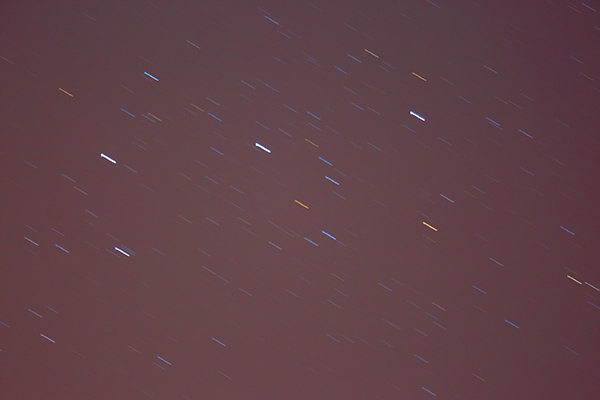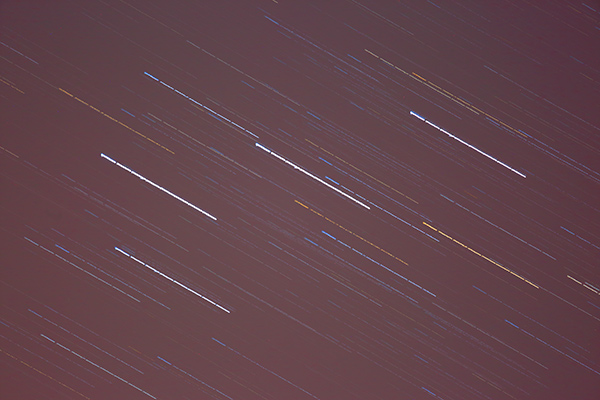# Jupiter properties

These notes supplement a talk I gave at Allenheads Observatory on 2017 April 7 about Jupiter and its moons.

## How to deduce some properties of Jupiter

This page describes how we can measure some important properties of Jupiter without sending spacecraft there. These methods can be applied to other planets too.

### The distance of Jupiter from the Sun

Kepler's 3rd law states that `P2 / a3` is a constant, the same for all planets (where `P` is the planet's orbital period and `a` is the semi-major axis of the elliptical orbit). If we assume that Jupiter's orbit is circular, which is almost true, then `a` is the radius of the circle.

We can easily measure the period `PJ` of Jupiter's orbit by seeing how long the planet takes to get back to the same position in the sky. It takes 11.9 (Earth) years. And of course `PE` (for the Earth) is exactly 1 year.

So `PJ2 / aJ3 = 11.92 / aJ3`

`=` (by Kepler) `PE2 / aE3 = 1.02 / aE3`

That can be evaluated to give `aJ = 5.2 aE`

So Jupiter is 5.2 times as far from the Sun as the Earth is. The Earth-Sun distance determines the "astronomical unit" (au) that is used for measuring all distances in the solar system.

Conclusion: Jupiter is 5.2au from the Sun.

First we need a method of measuring small angles in the sky.

The Earth rotates once (360 degrees) against the stars in 23 hours 56 minutes - the sidereal day. this corresponds to 15.04 degrees per hour or 1 degree in 3.99 minutes.

So, on a fixed mount (switch motors off), photograph a star trailing for 4 minutes to see what 1 degree amounts to for a given lens (or telescope). Then we can measure other objects in photos taken with that lens.

To keep it simple, use a star near the celestial equator, such as one of the belt stars in Orion (otherwise there is a factor of the cosine of the declination).

In a telescope we can use such a technique to measure the angle subtended by Jupiter, which turns out to be 50.1 arcseconds at opposition (0.0139 degrees).

We know how far Jupiter is from us (4.2au at opposition). So a little triangle geometry gives the true equatorial diameter of Jupiter as 71,500km or 11.2 x Earth.

`rJ = 11.2 rE`

There is an example at the bottom of this page showing how I made this measurement myself.

### The mass of Jupiter

Newton was able to explain Kepler's laws after he had deduced the formula for the gravitational force `F` between two masses `M` and `m` separated by distance `d`:

`F = G M m / d2` where `G` is a constant value that has been determined experimentally.

Combining that with Kepler's 3rd law gives (after some algebra, beyond the scope of this page)

`P2 / a3 = G (M + m) / 4π`

The right hand side of this equation was Kepler's constant in the case of planets orbiting the Sun but this equation is more general. It applies to any 2 masses orbiting each other, such as a moon orbiting a planet, in which case the planet's mass `M` is very much greater than a moon's mass `m`. In the case of Jupiter we can assume that the mass of any of the 4 Galilean moons is negligible compared to the mass of the planet. So, taking the moon Io as an example,

`PIo2 / aIo3 = G MJ / 4π`

By observing Io* to determine its orbital period and (by the method of the previous section) its orbital radius we can determine the mass `MJ` of Jupiter. It turns out to be 318 times the mass of the Earth:

`MJ` = 318 `ME`

* In practice accuracy could be improved by measuring all 4 of the moons.

### Jupiter's density

Density is defined as mass per unit volume:

`D = M / V`

So far we know that mass `MJ = 318 ME`

and radius `rJ = 11.2 rE`

But volume scales as radius cubed, so

`VJ = 11.23 VE = 1405 VE`

Density:

`DJ = MJ / VJ = 318 ME / 1405 VE = 0.266 DE`

The Earth is made of rock, iron, etc: `DE = 5.51 gm/cm3`

So `DJ = 1.25 gm/cm3` - very low!

The density is so low that when a mathematical model is made, taking account of increasing density with depth, it turns out that Jupiter must be made mostly of the lightest element, hydrogen. We were able to deduce this before any spacecraft were sent there but this conclusion has now been confirmed at close quarters.

### My example of measuring Jupiter's radius

This method can be applied to measuring other small angles in the sky.

On a fixed tripod I took a half-minute photo of Orion's belt (f/5.6 ISO800 32s 400mm lens on Canon 5D3):In fact I took 8 such photos in fairly quick succession and used my GRIP software to stack them together:You can just see short gaps between the exposures. By looking at the metadata (information) in the first and last image files I could see the timestamps. I took the difference between the two and added 32 seconds (although the camera says 30s on the dial it does in fact take 32s). So I know that the time difference between the beginning and end of each 8-image trail was 274 seconds. Now, due to the rotation of the Earth that is equivalent to 1.145 degrees.

So I was able to use GRIP to calibrate: the number of pixels in the image corresponding to 1 degree with this optical set-up. Then I photographed Jupiter with the same set-up and measured the diameter of Jupiter in degrees. More details of using GRIP to do that can be found here....

The answer I got was about 10% too large but that was because in a 400mm lens Jupiter is not many pixels across and also I rather over-exposed it. Using this technique in a telescope, with greater magnification, would be more practical.